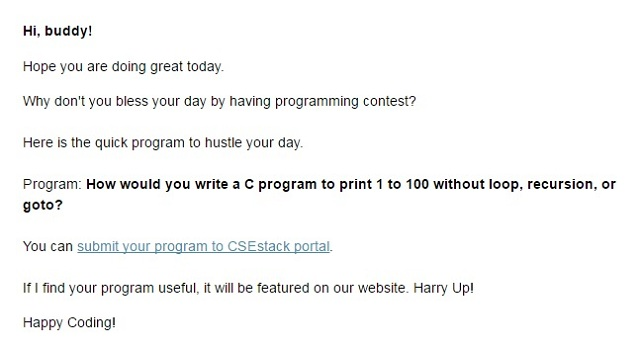# Write a C Program to Print 1 to 100 without Loop, Recursion or Goto

If you read like writing a C/C++ program to print 1 to 100, it’s so much easy. Right?

I am sure everyone here can write a program to print a series of numbers using loop, recursion or goto statement.

But what about if you are asked to write a C/C++ program to print 1 to 100 without loop, recursion, or goto?

First question, Is it possible to do that?

Yes, it is.

So here you go.

## C Program to Print 1 to 100 without Loop and Recursion:

The code is really simple but kind of tricky.

#### 1. Using Class Constructor

The trick is to create 100 objects, and each object will print one value.

• Write a class.
• Create 100 objects of the class. (If you create the array of the object, you don’t need to use loop for each object.)
• Write a constructor function in the class.
• Print the value for each object.
• Increment the value for each object by one.

Every call to constructor should write the different (incremental) value. You can do this by using a global variable. Each time it should call the constructor and increment the global variable by one. Then print the value.

Below is complete C++ code to program to print 1 to 100 without loop.

```#include <iostream>
using namespace std;

int i=0;

class S
{
public:
S()
{
i++;
cout<<i<<endl;
}
};

int main()
{
S A;
return 0;
}
```

You can print values in any given range.

You can initialize the global variable ‘i’ by any value which will be the first value in the range.
The number of objects of the class depicts total range.

There is also another solution by one of CSEstack subscriber.

#### 2. Using Template

If you are in CSEstack subscriber list, you might have received an email where I asked this question as a part of quick contest.Not so surprised, I got a really good response from the many programmers. It’s not possible to post all the answers, so I have picked something unique code from all replies.

Here is C++ code by Jeviravikumar(GV).

```#include <iostream>
using namespace std;

template<int N>
class PrintOneToN
{
public:
static void print()
{
PrintOneToN<N-1>::print();
// Note that this is not recursion
cout << N << endl;
}
};

template<>
class PrintOneToN<1>
{
public:
static void print()
{
cout << 1 << endl;
}
};
int main()
{
const int N = 100;
PrintOneToN<N>::print();
return 0;
}
```

Implementation is the same as an earlier program by creating N number of objects. Here, he has used the template as a generic data type.

This is the way you can print all the values from 1 to 100 without any recursion or loop. Many big tech companies ask this kind of tricky questions in their placement interviews.

Let’s take another quiz: Find Next Greatest Number with the Same Set of Digits.

This is the simple but tricky code program to print 1 to 100 without loop and recursion. I will keep sharing this kind of tricky coding questions. Subscribe to our channel and Stay tuned!